# SAT Math Multiple Choice Question 195: Answer and Explanation

### Test Information

Question: 195

15. At Santa Monica High School, the ratio of juniors to seniors is 4 to 3, the ratio of seniors to sophomores is 5 to 4, and the ratio of freshmen to sophomores is 7 to 6. What is the ratio of freshmen to seniors?

• A.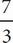• B.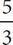• C.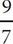• D.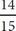D A good approach to this question would be to plug in some numbers. Start with the number of juniors and plug in a number that is easy to deal with, such as 200. Then you can set up a proportion using the ratio given in the question stem: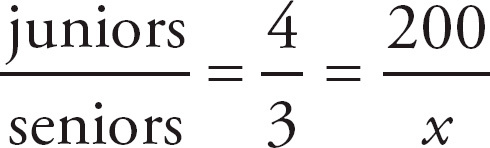. Cross-multiply and solve for x to determine that the number of seniors would be 150. Next, use the relationship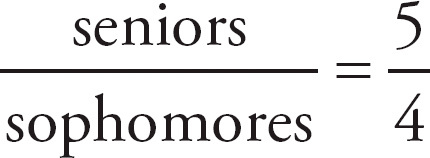. Using 150 for seniors, you get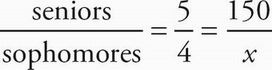. Cross-multiply and solve for x to determine that the number of sophomores would be 120. Next, use the relationship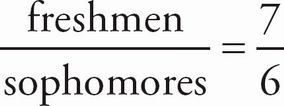. Using 120 for sophomores, you get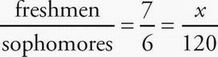. Cross-multiply and solve for x to determine that the number of freshmen would be 140. The ratio of freshmen to seniors, therefore, would be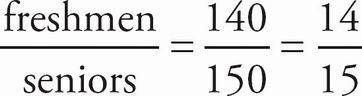, which is (D).